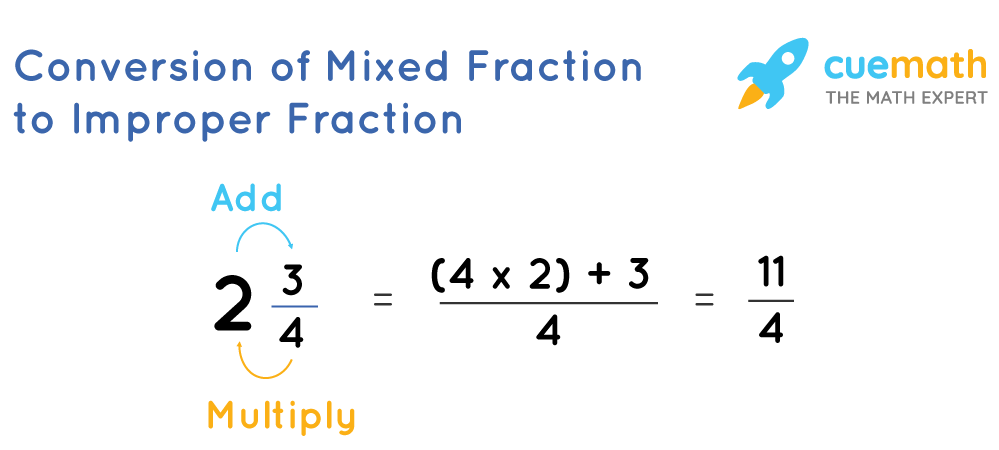# How to make a mixed number into a improper fraction

## Question: How to make a mixed number into an improper fraction?

A fraction in which the numerator is equal to or greater than the denominator is called an improper fraction.

A fraction which is a combination of a whole number and a proper fraction is known as a mixed fraction.

## Answer: To convert a mixed fraction into an improper fraction the whole number is multiplied with the denominator and the result is added to the numerator which becomes the numerator of the improper fraction. The denominator remains the same.

To turn a mixed fraction into an improper fraction, the following steps are followed.

## Explanation:

• Multiply the whole number with the denominator of the fractional part of the mixed number.
• Add the result to the numerator of the fractional part of the mixed number.
• This number obtained becomes the numerator of the improper fraction.
• The denominator of the improper fraction will be the same as the denominator of the fractional part of the mixed number.

Below is an example that shows the conversion of a mixed number to an improper fraction.